Win up to 100% scholarship on Aakash BYJU'S JEE/NEET courses with ABNAT Win up to 100% scholarship on Aakash BYJU'S JEE/NEET courses with ABNAT

# JEE Main 2022 July 28 – Shift 2 Chemistry Question Paper with Solutions

The JEE Main 2022 July 28 – Shift 2 Chemistry Question Paper with Solutions is provided on this page. Learning the JEE Main 2022 question paper with solutions will help students to make their knowledge stronger and thus score better ranks in the exam. Using the JEE Main 2022 answer keys, they can also verify their answers. They are recommended to revise JEE Main 2022 question papers so that they can improve their speed and accuracy. Check out the JEE Main 2022 July 28 – Shift 2 Chemistry Question Paper with Solutions given below.

## JEE Main 2022 28th July Shift 2 Chemistry Question Paper and Solutions

SECTION – A

Multiple Choice Questions: This section contains 20 multiple choice questions. Each question has 4 choices (1), (2), (3) and (4), out of which ONLY ONE is correct.

1. Given below are two statements: One is labelled as Assertion A and the other is labelled as Reason R

Assertion A: Zero orbital overlap is an out of phase overlap.

Reason R: It results due to different orientation/direction of approach of orbitals.

In the light of the above statements, choose the correct answer from the options given below

(A) Both A and R are true and R is the correct explanation of A

(B) Both A and R are true but R is NOT the correct explanation of A

(C) A is true but R is false

(D) A is false but R is true

Zero overlapping is something in which there is no overlapping between two orbitals. The first condition is that the two orbitals should not be symmetrical and the second condition is that both orbitals should be in different planes.

2. The correct decreasing order for metallic character is

(A) Na > Mg > Be > Si > P

(B) P > Si > Be > Mg > Na

(C) Si > P > Be > Na > Mg

(D) Be > Na > Mg > Si > P

Metallic character increases top to bottom in group and decreases left to right in a period.

Mg is from second group it will be less metallic than Na. Be comes above Mg hence less metallic than Mg. Si is more metallic than phosphorous.

3. Given below are two statements: One is labelled as Assertion A and the other is labelled as Reason R

Assertion A: The reduction of a metal oxide is easier if the metal formed is in liquid state than solid state.

Reason R: The value of ΔG becomes more on negative side as entropy is higher in liquid state than solid state.

In the light of the above statements, choose the most appropriate answer from the options given below

(A) Both A and R are correct and R is the correct explanation of A

(B) Both A and R are correct but R is not the correct explanation of A

(C) A is correct but R is not correct

(D) A is not correct but R is correct

Reduction of a metal oxide is easier if the metal is formed in a liquid state at the temperature of reduction because the entropy is higher if the metal is in a liquid state.

4. The products obtained during treatment of hard water using Clark’s method are:

$$\begin{array}{l} \left(A\right) CaCO_3 ~\text{and}~ MgCO_3\\ \left(B\right)Ca\left(OH\right)_2 ~\text{and} ~Mg(OH)_2\\ \left(C\right)CaCO_3 ~\text{and}~ Mg\left(OH\right)_2\\ \left(D\right)Ca\left(OH\right)_2 ~\text{and}~ MgCO_3\end{array}$$

Clark’s method :

$$\begin{array}{l} Mg\left(HCO_3\right)_2+2Ca\left(OH\right)_2\xrightarrow[~~~~]{}2CaCO_3\downarrow +Mg\left(OH_2\right)\downarrow +2H_2O\end{array}$$

5. Statement-I: An alloy of lithium and magnesium is used to make aircraft plates.

Statement-II: The magnesium ions are important for cell-membrane integrity.

In the light of the above statements, choose the correct answer from the options given below:

(A) Both Statement-I and Statement-II are true

(B) Both Statement-I and Statement-II are false

(C) Statement-I is true but Statement-II is false

(D) Statement-I is false but Statement-II is true

Magnesium alloys are used to make body of aircraft which is lightweight and resistant to corrosion.

Calcium is responsible for cell membrane integrity.

6. White phosphorus reacts with thionyl chloride to give

$$\begin{array}{l}\left(A\right) PCl_5, SO_2 ~\text{and}~ S_2Cl_2\\ \left(B\right) PCl_3, SO_2 ~\text{and}~S_2Cl_2\\ \left(C\right) PCl_3, SO_2 ~\text{and}~Cl_2\\ \left(D\right) PCl_5, SO_2 ~\text{and}~Cl_2\end{array}$$

Sol

$$\begin{array}{l} \underset{\text{White phosphorous}}{P_4}+\underset{\text{Thionyl chloride}}{8SOCl_2}\xrightarrow[~~~]{}4PCl_3+4SO_2+2S_2Cl_2\end{array}$$

7. Concentrated HNO3 reacts with Iodine to give

$$\begin{array}{l}\left(A\right) Hl, NO_2 ~\text{and}~ H_2O\\ \left(B\right) HlO_2, N_2O ~\text{and}~ H_2O\\ \left(C\right) HlO_3, NO_2 ~\text{and}~ H_2O\\ \left(D\right) HlO_4, N_2O ~\text{and}~ H_2O\end{array}$$

Sol

$$\begin{array}{l} I_2 + 10HNO_3 \rightarrow 2HIO_3 + 10NO_2 + 4H_2O\end{array}$$

8. Which of the following pairs is not isoelectronic species?

(At. no. Sm, 62; Er, 68; Yb, 70; Lu, 71; Eu, 63; Tb, 65; Tm, 69)

(A) Sm2+ and Er3+

(B) Yb2+ and Lu3+

(C) Eu2+ and Tb4+

(D) Tb2+ and Tm4+

Sol Species having same number of electrons are isoelectronic

$$\begin{array}{l} \left.\begin{matrix}Tb\xrightarrow[~~~]{}65 & Tb^{2+}\xrightarrow[~~~]{}63\text{ electrons} \\Tm\xrightarrow[~~]{}69 & Tm^{4+}\xrightarrow[~~~]{}65\text{ electrons} \\\end{matrix}\right\}\text{not isoelectronic}\end{array}$$
$$\begin{array}{l} \left.\begin{matrix}Sm^{2+}\xrightarrow[~~~]{}60\text{ electrons} \\Er^{3+}\xrightarrow[~~~]{}65\text{ electrons}\end{matrix}\right\}\text{ not isoelectronic}\end{array}$$

9. Given below are two statements: One is labelled as Assertion A and the other is labelled as Reason R.

Assertion A: Permanganate titrations are not performed in presence of hydrochloric acid.

Reason R: Chlorine is formed as a consequence of oxidation of hydrochloric acid.

In the light of the above statements, choose the correct answer from the options given below.

(A) Both A and R are true and R is the correct explanation of A

(B) Both A and R are true but R is NOT the correct explanation of A

(C) A is true but R is false

(D) A is false but R is true

Sol HCl is not used in the process of titration because it reacts with the (KMnO4) that is used in the process and gets oxidized.

10. Match List-I with List-II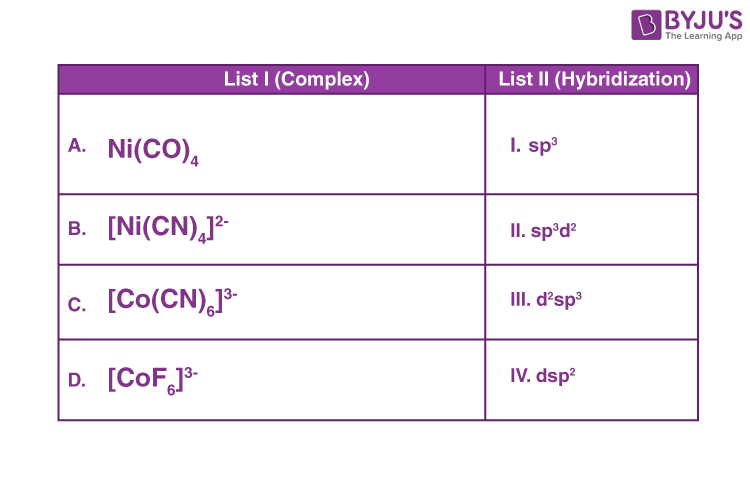Choose the correct answer from the options given below:

(A) A-IV, B-I, C-III, D-II

(B) A-I, B-IV, C-III, D-II

(C) A-I, B-IV, C-II, D-III

(D) A-IV, B-I, C-II, D-III

Sol A. Ni(CO)4→ sp3

B [Ni(CN)4]–2→ dsp2

C. [Co(CN)6]–3→ d2sp3

D. [CoF6]–3→ sp3d2

11. Dinitrogen and dioxygen, the main constituents of air do not react with each other in atmosphere to form oxides of nitrogen because

(A) N2 is unreactive in the condition of atmosphere

(B) Oxides of nitrogen are unstable

(C) Reaction between them can occur in the presence of a catalyst

(D) The reaction is endothermic and require very high temperature

Sol. N2 is unreactive, its reaction with oxides is endothermic and require very high temperature.

12. The major product in the given reaction is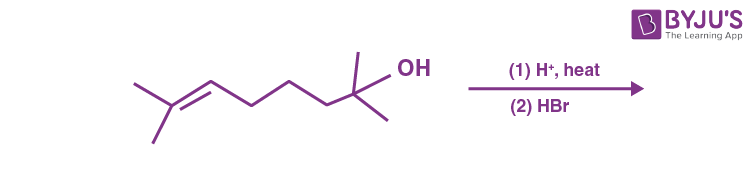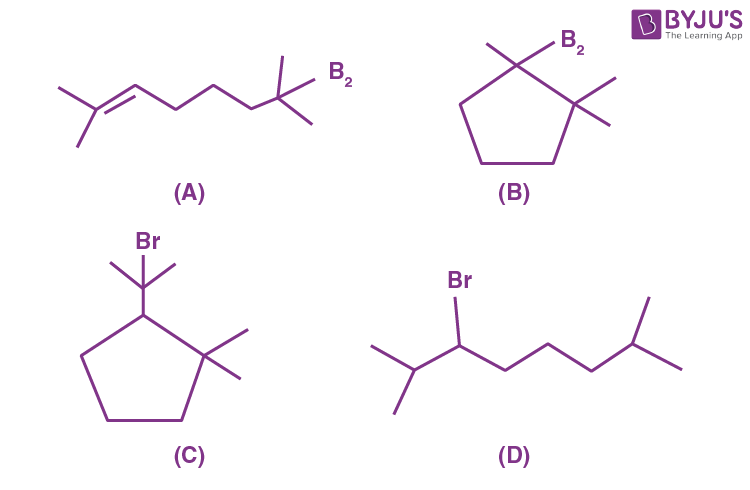Sol.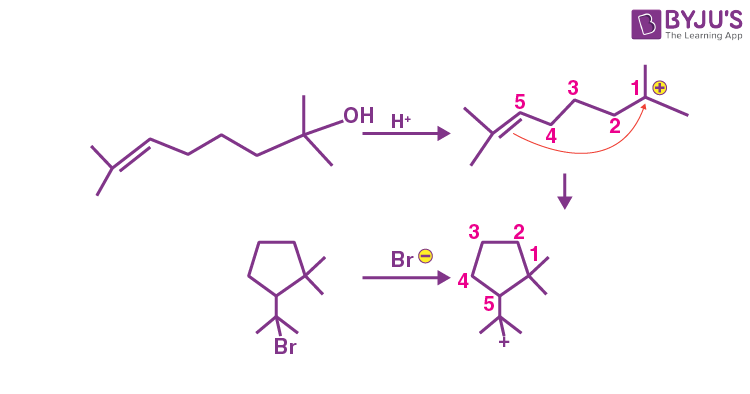13. Arrange the following in increasing order of reactivity towards nitration

A. p-xylene

B. bromobenzene

C. mesitylene

D. nitrobenzene

E. benzene

(A) C < D < E < A < B

(B) D < B < E < A < C

(C) D < C < E < A < B

(D) C < D < E < B < A

Sol. The correct order of reactivity towards nitration is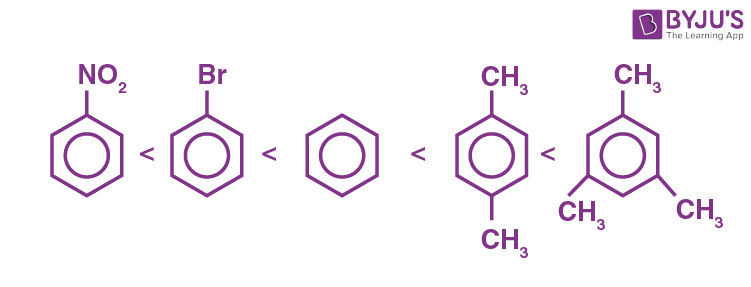as electron releasing groups on benzene ring facilitate the nitration at benzene ring.

14. Compound I is heated with Conc. HI to give a hydroxy compound A which is further heated with Zn dust to give compound B. Identify A and B.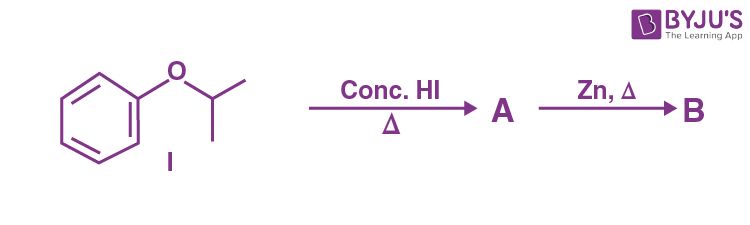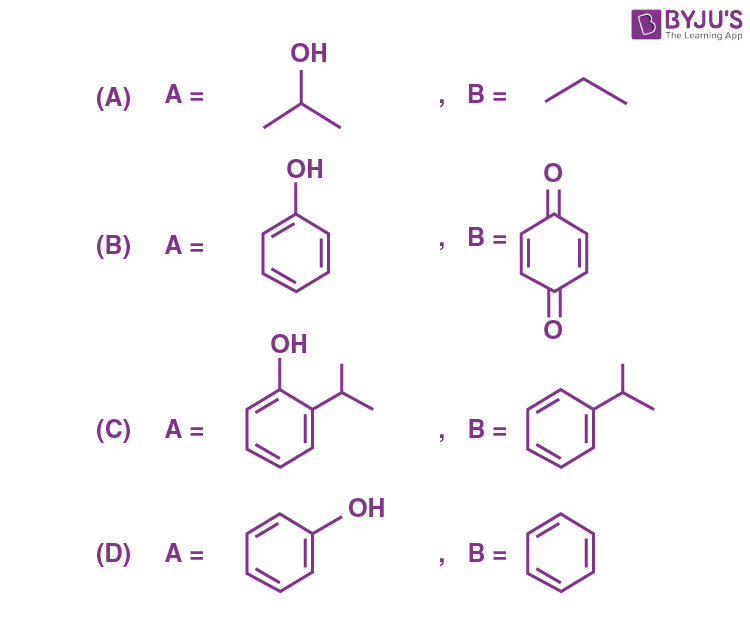Sol.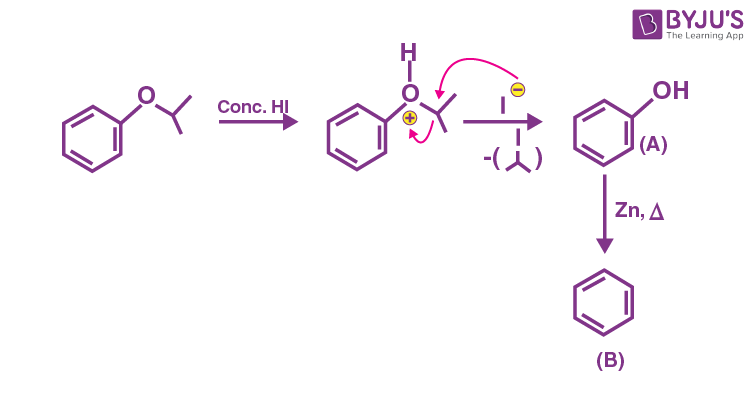15. Given below are two statements: one is labelled as Assertion A and the other is labelled as Reason R

Assertion A : Aniline on nitration yields ortho, meta & para nitro derivatives of aniline.

Reason R : Nitrating mixture is a strong acidic mixture.

In the light of the above statements, choose the correct answer from the options given below.

(A) Both A and R are true and R is the correct explanation of A

(B) Both A and R are true but R is NOT the correct explanation of A

(C) A is true but R is false

(D) A is false but R is true

Sol.16. Match List I with List II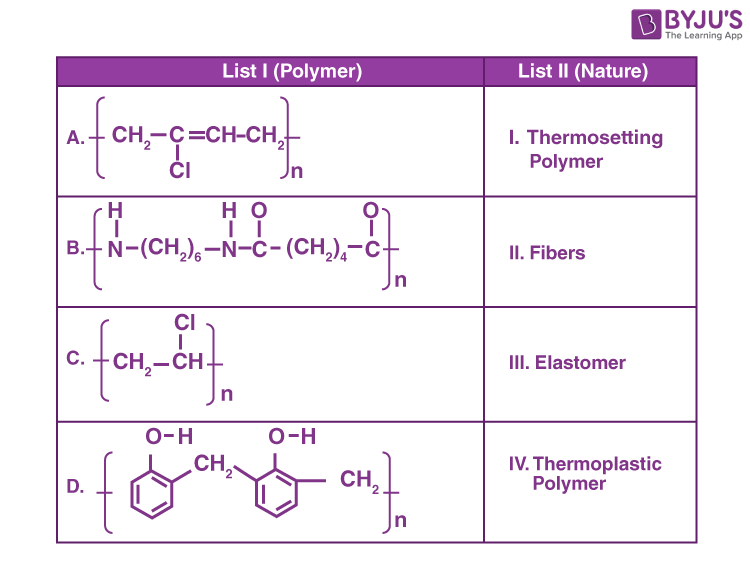Choose the correct answer from the options given below:

(A) A-II, B-III, C-IV, D-I

(B) A-III, B-II, C-IV, D-I

(C) A-III, B-I, C-IV, D-II

(D) A-I, B-III, C-IV, D-II

Sol.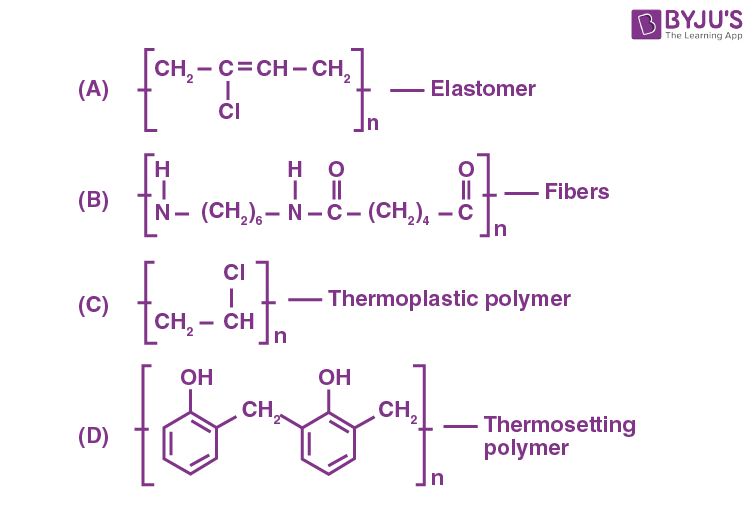17. Two statements in respect of drug-enzyme interaction are given below

Statement I: Action of an enzyme can be blocked only when an inhibitor blocks the active site of the enzyme.

Statement II: An inhibitor can form a strong covalent bond with the enzyme.

In the light of the above statements, choose the correct answer from the options given below

(A) Both Statement I and Statement II are true

(B) Both Statement I and Statement II are false

(C) Statement I is true but Statement II is false

(D) Statement I is false but Statement II is true

Sol. Action of an enzyme can be altered by a number of factors like temperature, pH, presence of activators and coenzymes and presence of inhibitors and poisons.

Inhibitors or poisons interact with the active functional groups on the enzyme surface and often reduce or completely destroy the catalytic activity of the enzymes.

18. Given below are two statements: One is labelled
as Assertion A and the other is labelled as
Reason R.

Assertion A: Thin layer chromatography is an adsorption chromatography.

Reason R: A thin layer of silica gel is spread over a glass plate of suitable size inthin layer chromatography which acts as an adsorbent.

In the light of the above statements, choose the correct answer from the options given below

(A) Both A and R are true and R is the correct explanation of A.

(B) Both A and R are true but R is NOT the correct explanation of A

(C) A is true but R is false

(D) A is false but R is true

Sol. Thin layer chromatography is an adsorption chromatography. A thin layer of silica gel is spreadover a glass plate of suitable size and act as an adsorbent.

19. The formulas of A and B for the following reaction sequence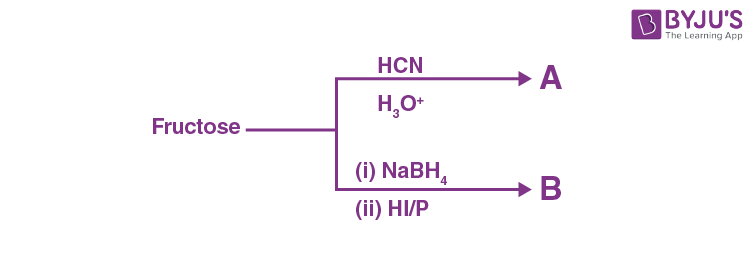are

(A) A = C7H14O8, B = C6H14

(B) A = C7H13O7, B = C7H14O

(C) A = C7H12O8, B = C6H14

(D) A = C7H14O8, B = C6H14O6

Sol.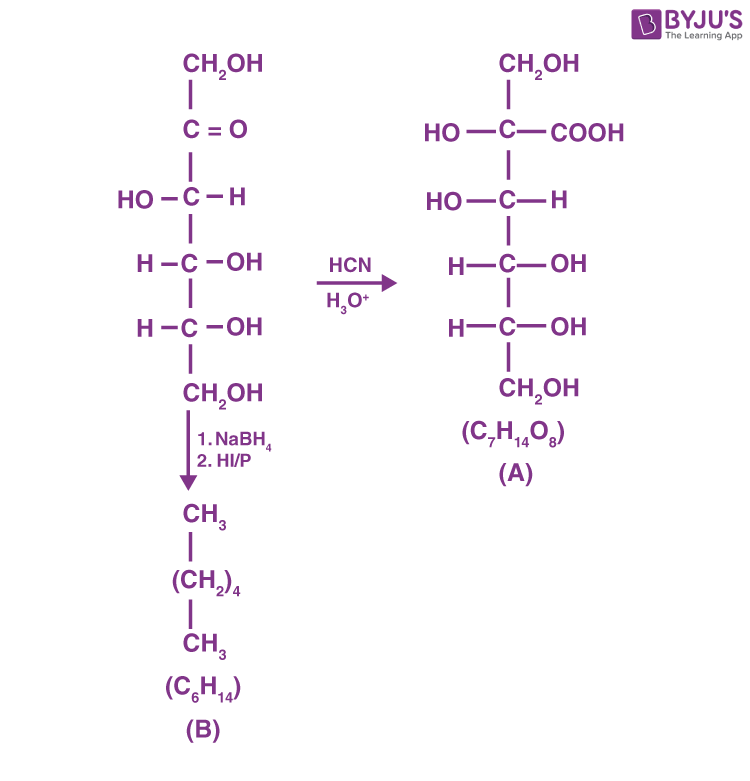20.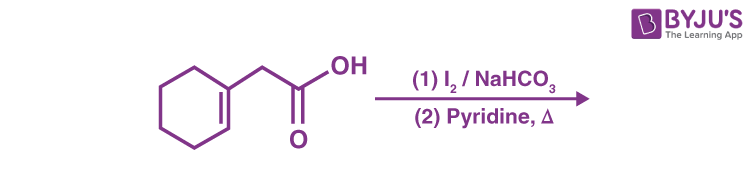Find out the major product for the above reaction.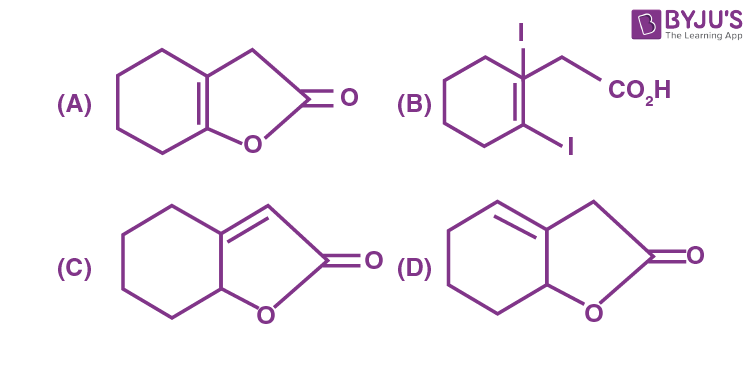Sol.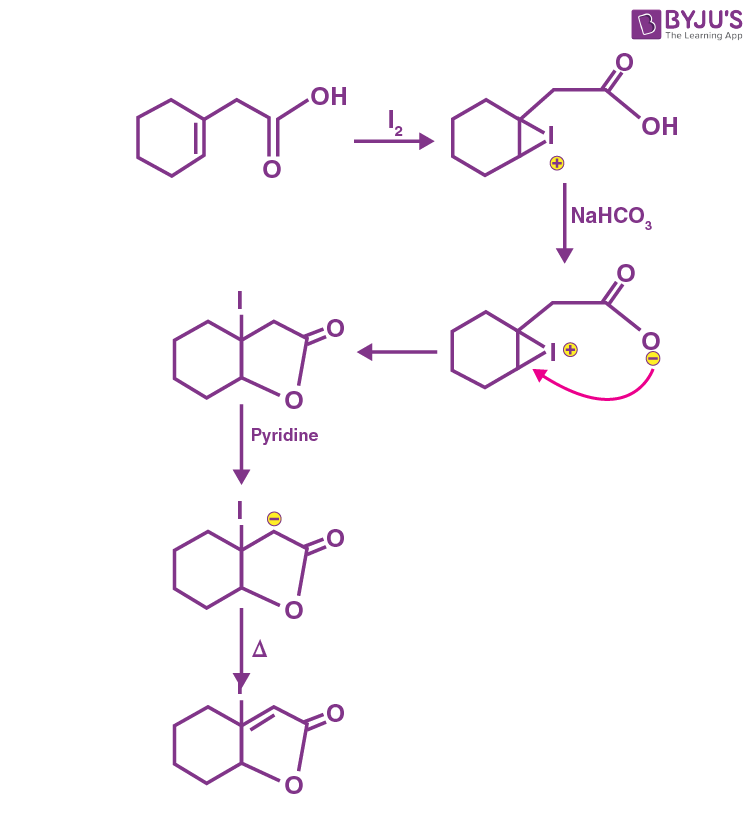SECTION – B

Numerical Value Type Questions: This section contains 10 questions. In Section B, attempt any five questions out of 10. The answer to each question is a NUMERICAL VALUE. For each question, enter the correct numerical value (in decimal notation, truncated/rounded-off to the second decimal place; e.g. 06.25, 07.00, –00.33, –00.30, 30.27, –27.30) using the mouse and the on-screen virtual numeric keypad in the place designated to enter the answer.

1. 2 L of 0.2 M H2SO4 is reacted with 2 L of 0.1 M NaOH solution, the molarity of the resulting product Na2SO4 in the solution is _______ millimolar. (Nearest integer)

Sol.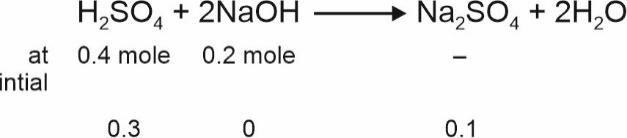$$\begin{array}{l} \text{ Molarity of }Na_2SO_4=\frac{0.1}{4}=0.025\text{ M}\\= 25~ \text{millimolar} \end{array}$$

2. Metal M crystallizes into a fcc lattice with the edge length of 4.0 × 10–8 cm. The atomic mass of the metal is _______ g/mol. (Nearest integer)

$$\begin{array}{l}\left(\text{Use} : N_A = 6.02 \times 10^{23}~ \text{mol}^{-1}, \text{density of metal}, M = 9.03~ \text{g cm}^{-3}\right)\end{array}$$

Sol.

$$\begin{array}{l} \rho=\frac{ZM}{N_Aa^3}\Rightarrow M=\frac{9.03\times 6.02\times 10^{23}\times\left(4\times10^{-8}\right)^3}{4} \end{array}$$
$$\begin{array}{l} =\frac{9.03\times6.02\times64\times10^{-1}}{4} \end{array}$$
$$\begin{array}{l}= 86.9 ~\text{g mol}^{-1}\\ \approx 87 ~\text{g mol}^{-1}\end{array}$$

3. If the wavelength for an electron emitted from H-atom is 3.3 × 10–10 m, then energy absorbed by the electron in its ground state compared to minimum energy required for its escape from the atom, is ______ times. (Nearest integer)

$$\begin{array}{l}\left[\text{Given} : h = 6.626 \times 10^{-34}~ \text{J s}\right]\\ \left[\text{Mass of electron} = 9.1 \times 10^{-31} ~\text{kg}\right]\end{array}$$

Sol.

$$\begin{array}{l} \lambda =\frac{h}{mv} \end{array}$$
$$\begin{array}{l} \Rightarrow mv=\frac{h}{\lambda}=\frac{6.626\times10^{-34}\text{kg}\frac{m^2}{\sec^2}\times\sec}{3.3\times10^{-10}\text{ m}}\end{array}$$
$$\begin{array}{l} mv=\frac{6.626\times10^{-24}}{3.3}=2\times 10^{-24}~\text{kg m}\sec^{-1}\end{array}$$
$$\begin{array}{l} \text{Kinetic energy}=\frac{1}{2}\text{ mv}^2 \end{array}$$
$$\begin{array}{l} =\frac{\left(mv\right)^2}{2m} \end{array}$$
$$\begin{array}{l} =\frac{\left(2\times10^{-24}\right)^2}{2\times9.1\times10^{-31}\text{kg}}\end{array}$$
$$\begin{array}{l}= 2.18 \times 10^{-18}\text{J}\\ = 21.8 \times 10^{-19} \text{J}\end{array}$$
$$\begin{array}{l} \begin{matrix}\text{Total energy} & = & \text{lonization} & + & \text{Kinetic} \\\text{absorbed} & & \text{energy} & &\text{energy} \\\end{matrix}\end{array}$$
$$\begin{array}{l}= \left(21.76 + 21.8\right) \times 10^{-19}\\ = 43.56 \times 10^{-19}~ \text{J}\\ \approx 2 ~\text{times of}~ 21.76 \times 10^{-19} ~\text{J} \end{array}$$

4. A gaseous mixture of two substances A and B, under a total pressure of 0.8 atm is in equilibrium with an ideal liquid solution. The mole fraction of substance A is 0.5 in the vapour phase and 0.2 in the liquid phase. The vapour pressure of pure liquid A is ______ atm. (Nearest integer)

Sol. Given that XA = 0.2, YA = 0.5, PT = 0.8 atm

We know that PA = YA × PT

PA = 0.5 × 0.8 = 0.4

Now

$$\begin{array}{l} P_A=X_A\times P_A^\circ \Rightarrow P_A^\circ=\frac{0.4}{0.2}=2\text{ atm}\end{array}$$

5. At 600 K, 2 mol of NO are mixed with 1 mol of O2.

$$\begin{array}{l}2NO\left(g\right)+O_2\left(g\right)\rightleftharpoons 2NO_2\left(g\right)\end{array}$$

The reaction occurring as above comes to equilibrium under a total pressure of 1 atm. Analysis of the system shows that 0.6 mol of oxygen are present at equilibrium. The equilibrium constant for the reaction is _______. (Nearest integer)

Sol.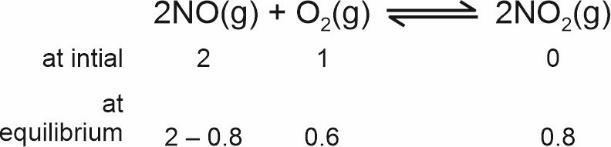$$\begin{array}{l} \text{Partial pressure of NO(g)}=\frac{1.2}{2.6}\times1\end{array}$$
$$\begin{array}{l} \text{Partial pressure of }O_2\left(g\right)=\frac{0.6}{2.6} \end{array}$$
$$\begin{array}{l} \text{Partial pressure of }NO_2\left(g\right)=\frac{0.8}{2.6} \end{array}$$
$$\begin{array}{l}K_p=\frac{\left(P_{NO_2}\right)^2}{\left(P_{NO}\right)^2\left(P_{O_2}\right)} =\frac{0.8\times0.8\times2.6}{1.2\times1.2\times0.6}\\ = 1.925\\ \approx 2 \end{array}$$

6. A sample of 0.125 g of an organic compound when analysed by Duma’s method yields 22.78 mL of nitrogen gas collected over KOH solution at 280 K and 759 mm Hg. The percentage of nitrogen in the given organic compound is____.(Nearest integer)

Given :

1. The vapour pressure of water of 280 K is 14.2 mm Hg.
2. R = 0.082 L atm K–1 mol–1

Sol.

$$\begin{array}{l}P_{actual} = 759 – 14.2 = 744.8 ~\text{mmHg}\end{array}$$
$$\begin{array}{l}n_{N_2}=\frac{744.8\times22.78}{760\times0.0821\times280\times1000}\\ = 0.000971 ~\text{mol}\end{array}$$

Mass of N2 = 0.02719 gm

$$\begin{array}{l}\text{Percentage of nitrogen} =\frac{0.0271}{0.125}\times100=21.75\simeq 22 \end{array}$$

7. On reaction with stronger oxidizing agent like KIO4, hydrogen peroxide oxidizes with the evolution of O2. The oxidation number of I in KIO4 changes to____.

Sol.

$$\begin{array}{l} \overset{+7}{KIO_4}+H_2O_2\xrightarrow[~~~~]{}\overset{+5}{KIO_3}+H_2O+O_2\end{array}$$

8. For a reaction, given below is the graph of ln k vs 1/T. The activation energy for the reaction is equal to____ cal mol–1. (Nearest integer)

(Given: R = 2 cal K-1 mol-1)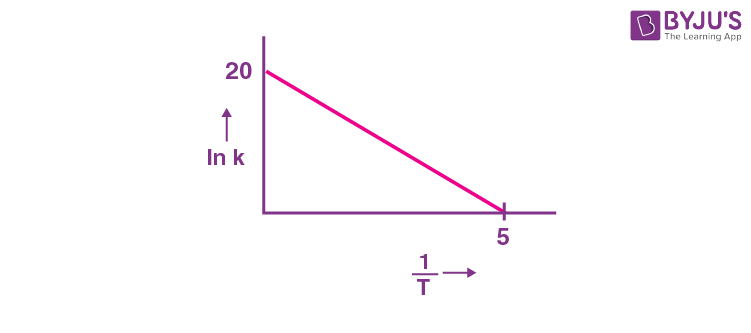Sol.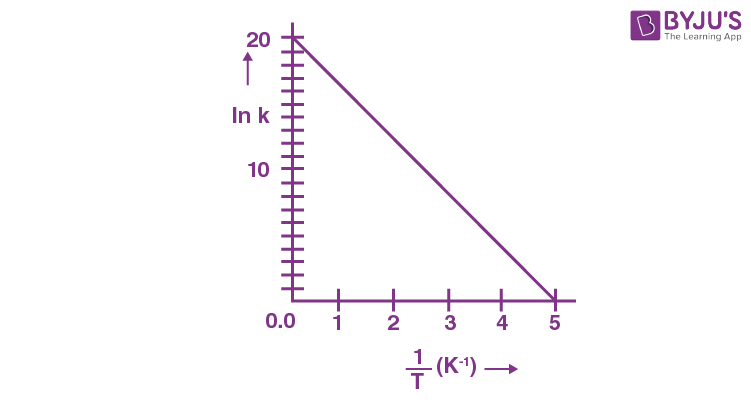$$\begin{array}{l} \text{Slope}=\frac{-20}{5}\end{array}$$
$$\begin{array}{l} \text{ ln }k=\text{ ln }A-\frac{E_a}{RT} \end{array}$$
$$\begin{array}{l} \therefore\ \frac{E_a}{R}=\frac{20}{5}\Rightarrow E=\frac{20 R}{5}=8 \text{ cal mol}^{-1}\end{array}$$

9. Among the following the number of curves not in accordance with Freundlich adsorption isotherm is____.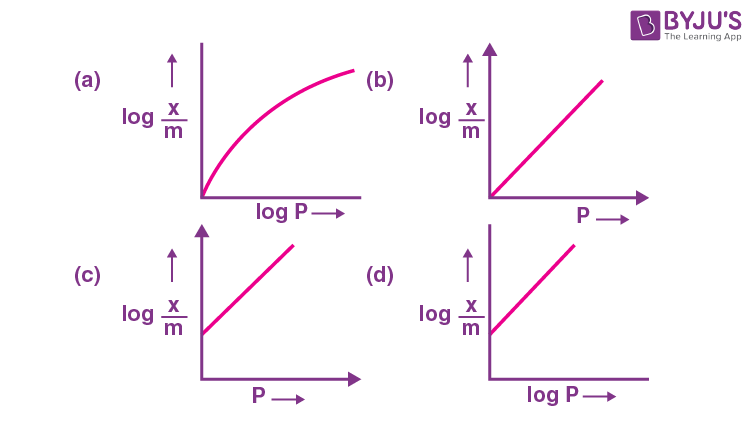Sol. The following curves are not in accordance with Freundlich adsorption isotherm.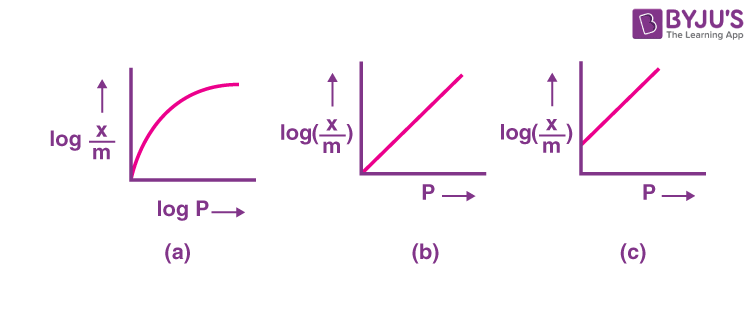10. Among the following the number of state variables is____.

Internal energy (U)

Volume (V)

Heat (q)

Enthalpy (H)

Sol. State variables are internal energy (U), Volume (V)

and Enthalpy (H).

### Download PDF of JEE Main 2022 July 28 Shift 2 Chemistry Paper & Solutions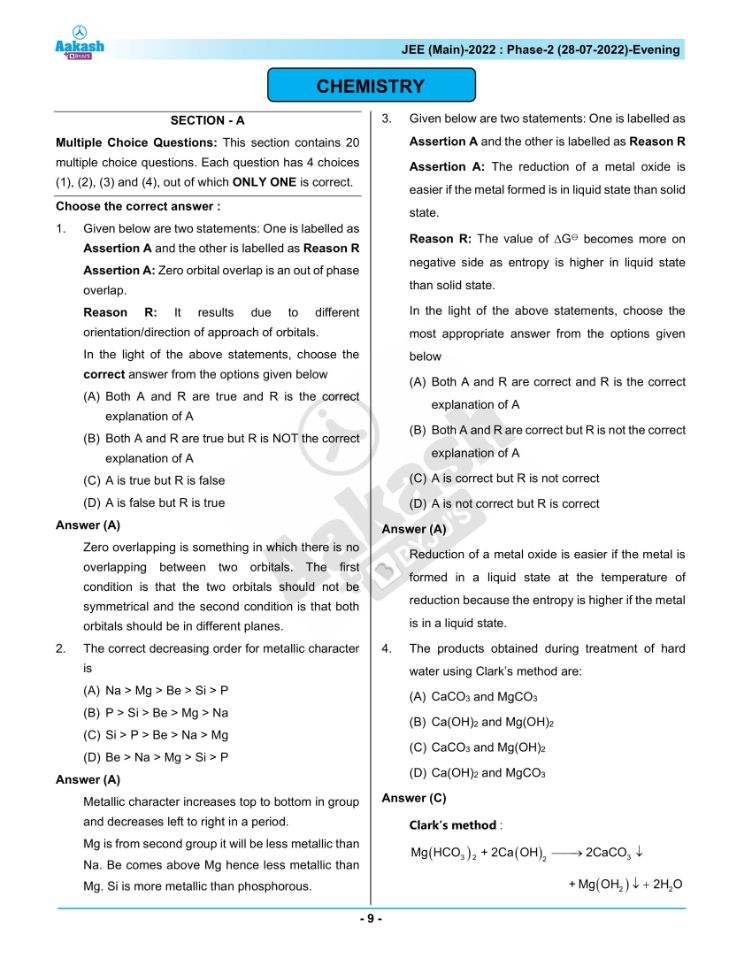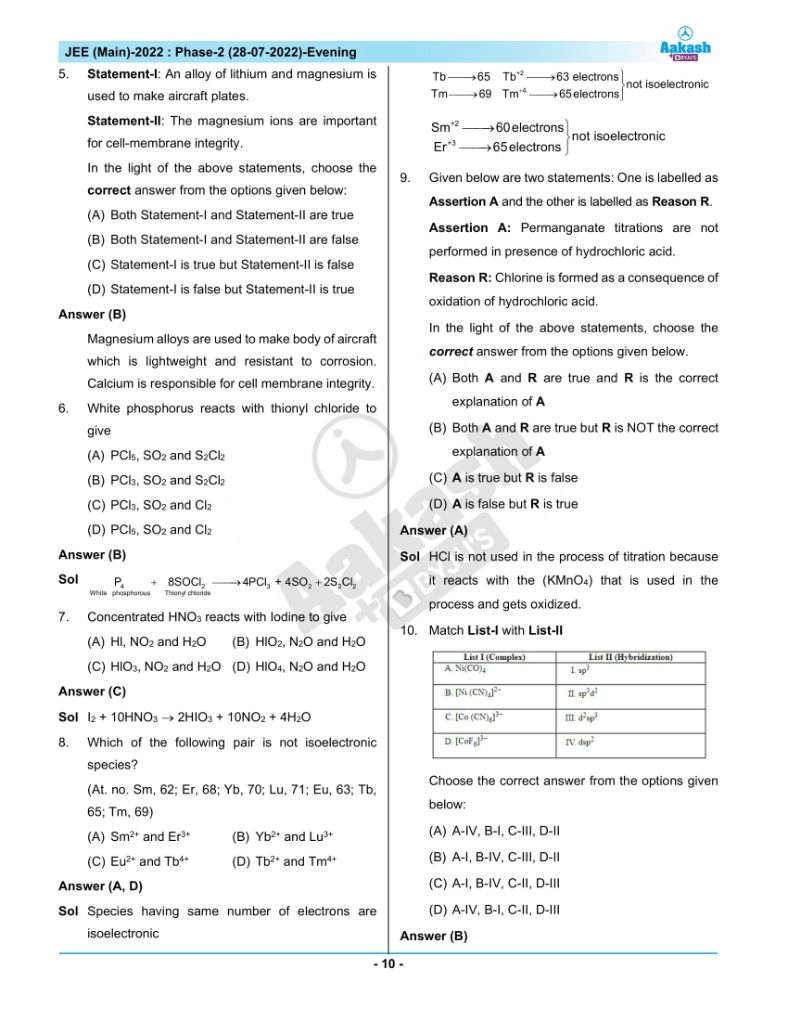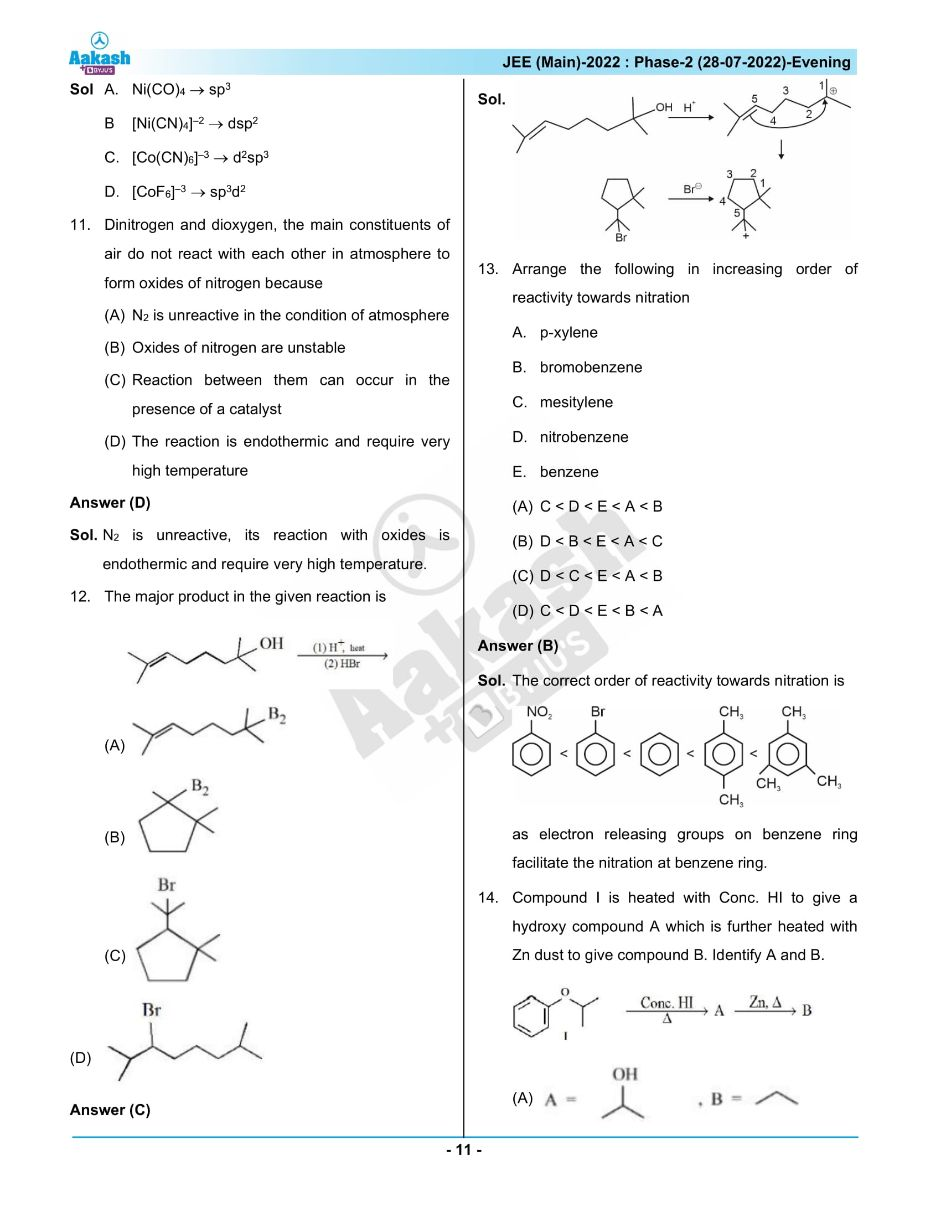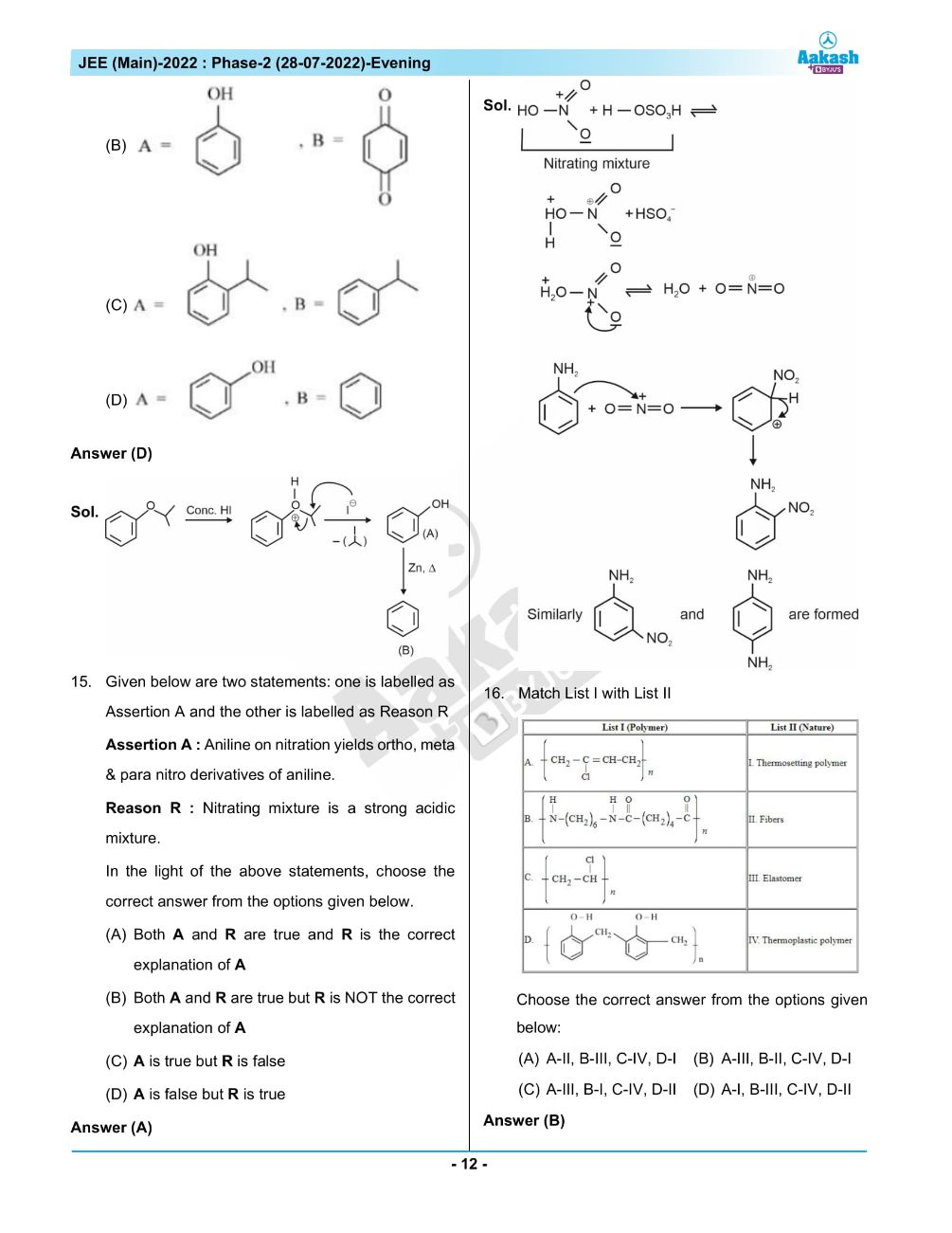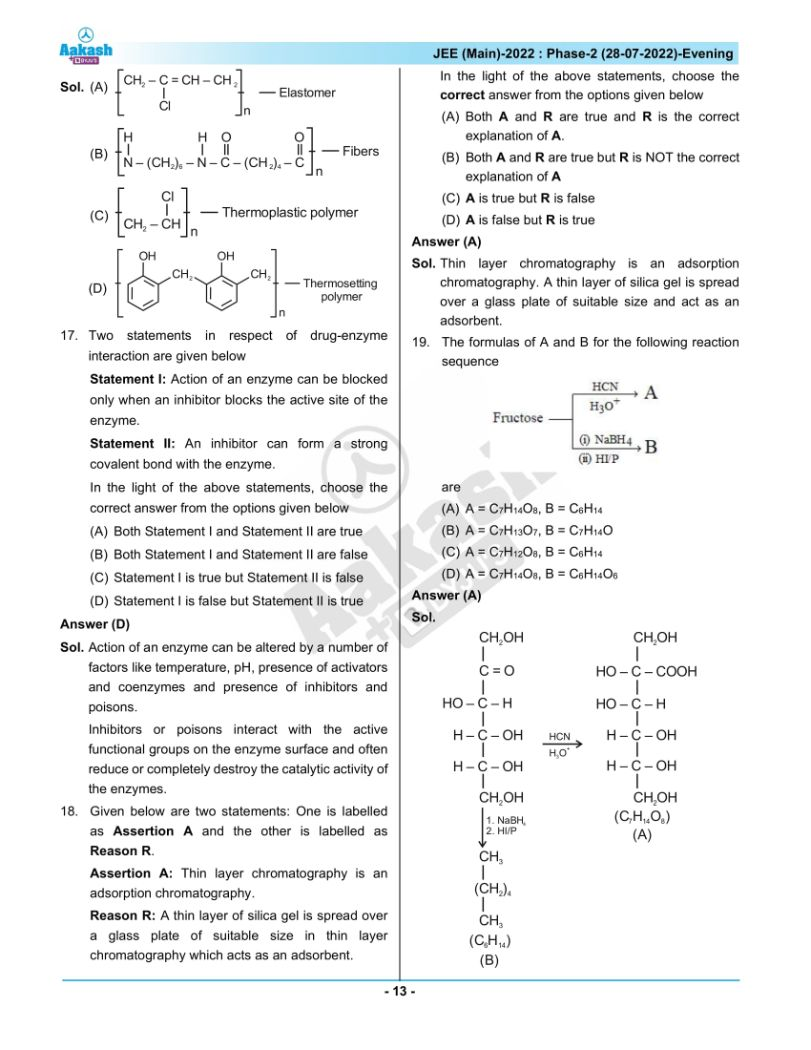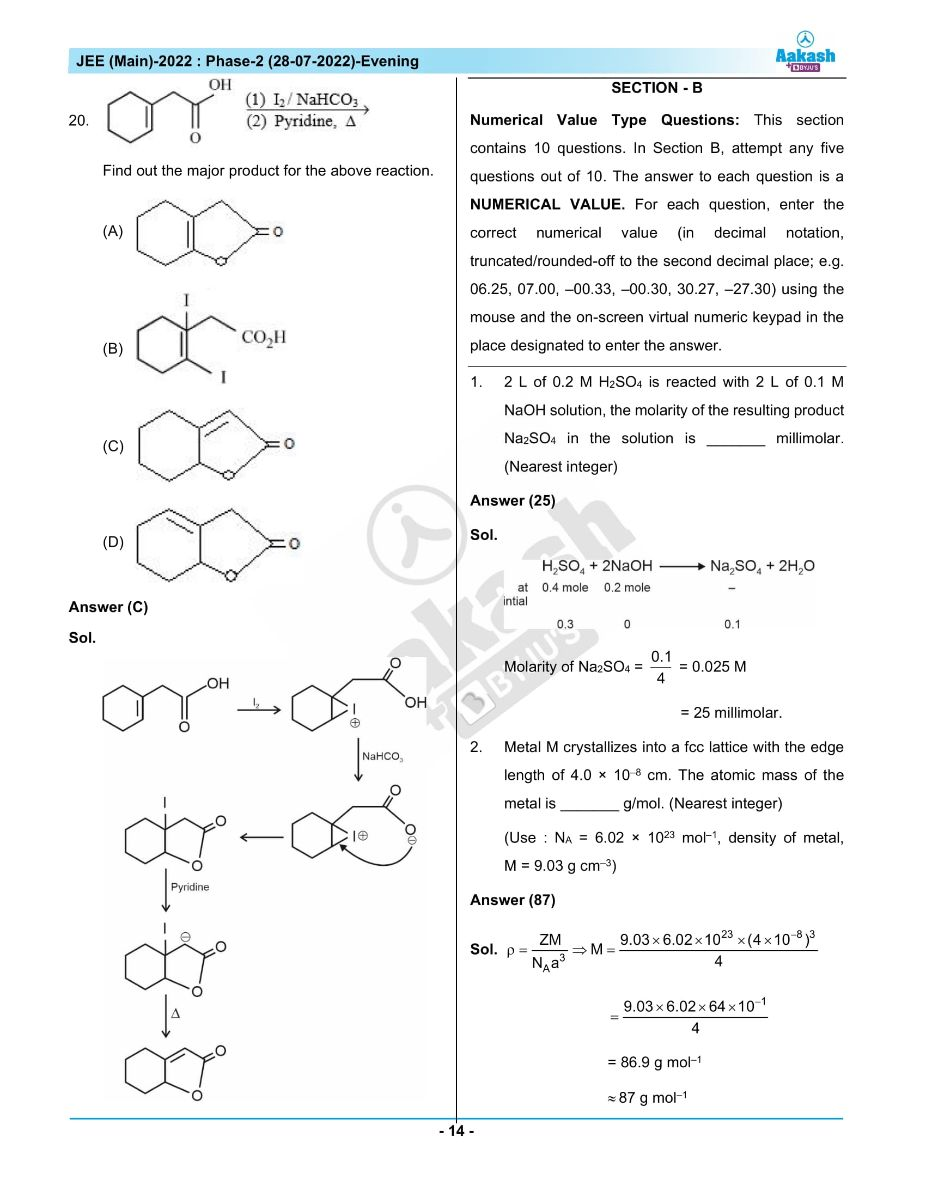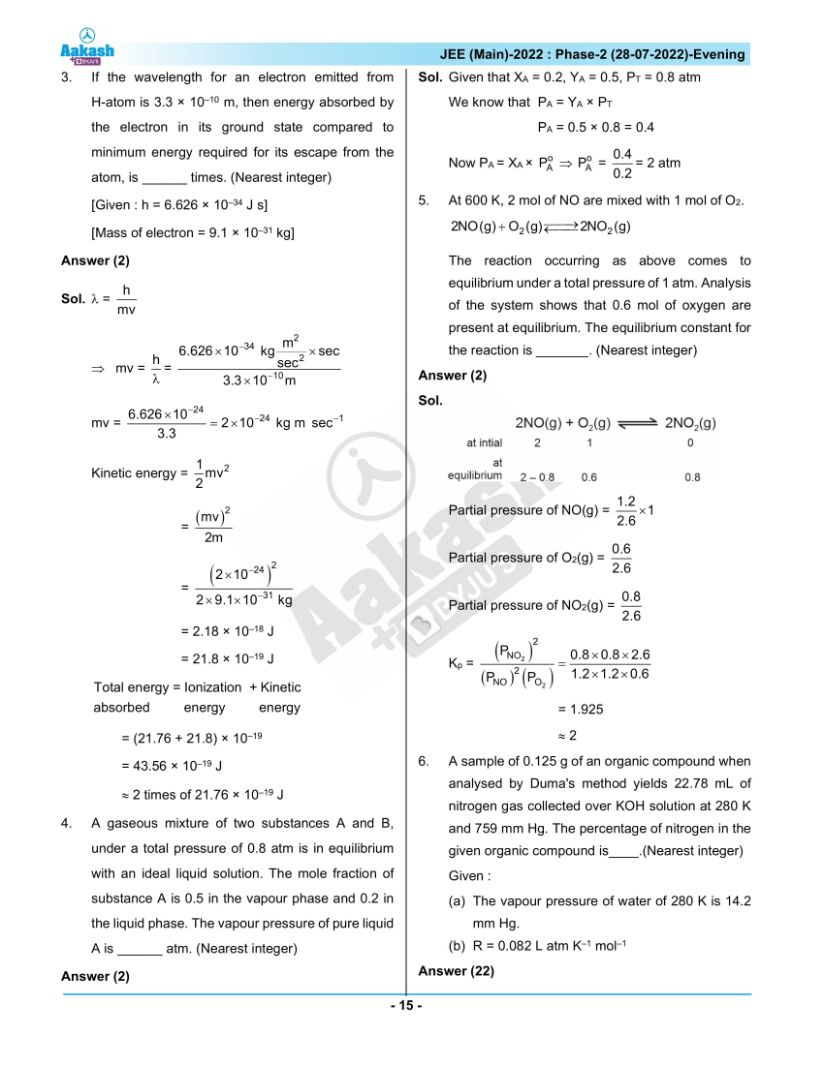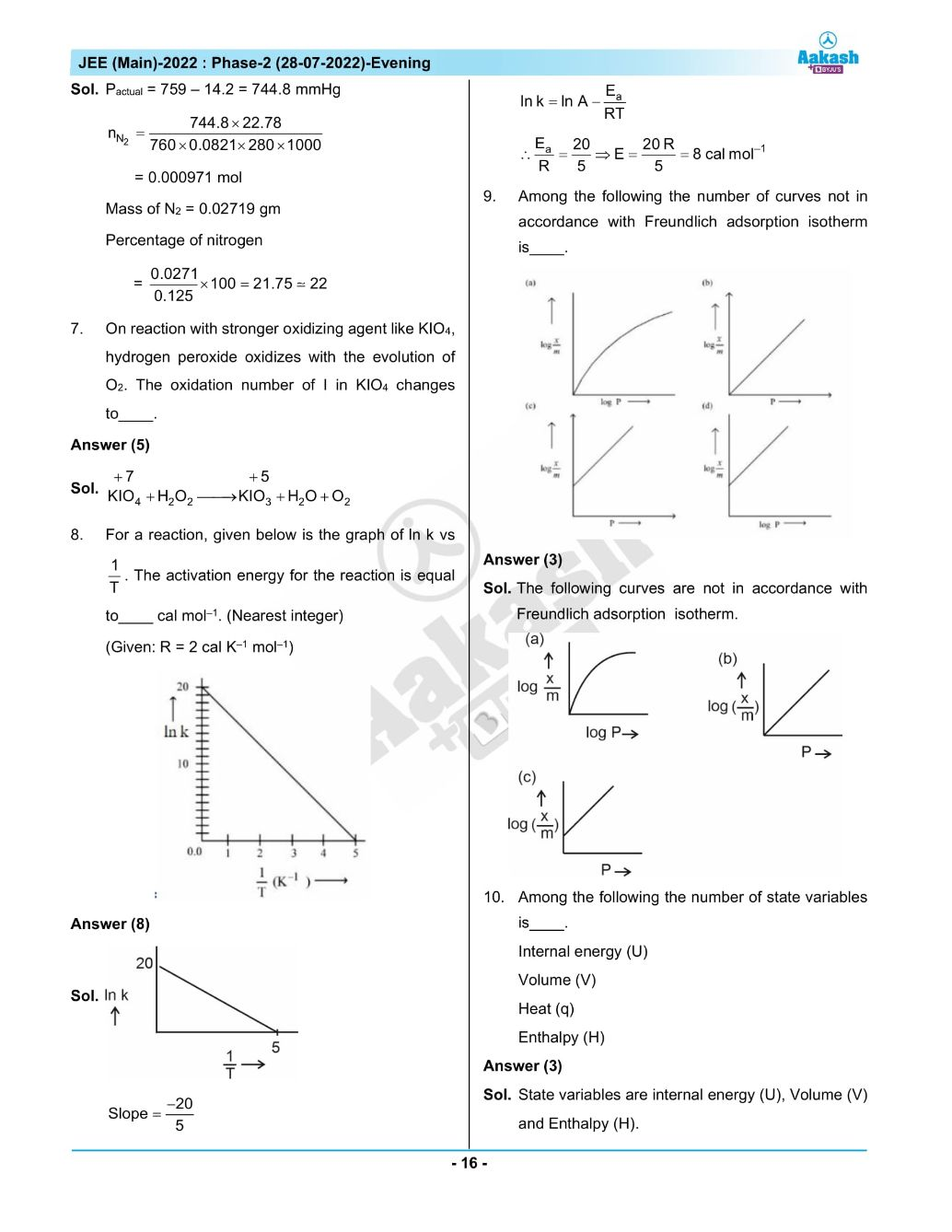## JEE Main 2022 July 28th Shift 2 Paper Analysis